### No Common Sense Shadow Art (Resubmited)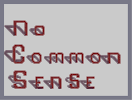Hover over the thumbnail for a full-size version.

Author no_common_sense author:no_common_sense autogenerated n-art rated 2006-10-02 2006-10-02 4 by 17 people. \$No Common Sense Shadow Art (Resubmited)#no_common_sense#none#00000000000000000000000000000000000000000000000000000000000000000000000000000000000000000000000000000000000000000000000000000000000000000000000000000000000000000000000000000000000000000000000000000000000000000000000000000000000000000000000000000000000000000000000000000000000000000000000000000000000000000000000000000000000000000000000000000000000000000000000000000000000000000000000000000000000000000000000000000000000000000000000000000000000000000000000000000000000000000000000000000000000000000000000000000000000000000000000000000000000000000000000000000000000000000000000000000000000000000000000000000000000000000000000000000000000000000000000000000000000000000000000000000000000000000000000000000000000000000|12^132,306!12^132,300!12^132,294!12^132,288!12^138,288!12^138,294!12^138,300!12^138,306!12^144,306!12^144,300!12^144,294!12^144,288!12^138,282!12^132,282!12^126,282!12^132,276!12^126,276!12^120,276!12^126,270!12^120,270!12^114,270!12^108,270!12^102,270!12^114,276!12^108,276!12^102,276!12^96,276!12^96,270!12^90,276!12^96,282!12^90,282!12^84,282!12^90,288!12^84,288!12^78,288!12^78,294!12^78,300!12^78,306!12^78,312!12^78,312!12^78,318!12^78,324!12^78,330!12^78,342!12^78,336!12^78,348!12^78,354!12^78,360!12^84,294!12^84,300!12^84,312!12^84,306!12^84,318!12^84,324!12^84,330!12^84,336!12^84,342!12^84,348!12^84,354!12^84,360!12^90,360!12^90,354!12^90,348!12^90,342!12^90,336!12^90,330!12^90,324!12^90,318!12^90,312!12^90,306!12^90,300!12^90,294!12^84,366!12^90,366!12^96,366!12^90,372!12^96,372!12^102,372!12^96,378!12^102,378!12^108,378!12^114,378!12^120,378!12^114,372!12^108,372!12^120,372!12^126,378!12^126,372!12^132,372!12^126,366!12^132,366!12^138,366!12^132,360!12^144,360!12^144,354!12^144,348!12^144,342!12^138,342!12^132,342!12^132,348!12^132,354!12^138,354!12^138,348!12^90,378!12^84,372!12^78,366!12^78,282!12^84,276!12^90,270!12^132,378!12^138,372!12^144,366!12^144,282!12^138,276!12^132,270!3^72,366!3^72,360!3^66,360!3^66,354!3^60,354!3^72,354!3^66,348!3^60,348!3^54,348!3^48,342!3^54,342!3^60,342!3^42,336!3^48,336!3^54,336!3^78,366!3^36,330!3^42,330!3^48,330!3^30,324!3^36,324!3^42,324!3^24,318!3^30,318!3^36,318!3^30,312!3^36,306!3^36,312!3^42,306!3^42,312!3^48,312!3^48,306!3^54,306!3^54,312!3^60,312!3^66,306!3^60,306!3^66,312!3^72,312!3^72,306!3^96,306!3^102,306!3^96,312!3^102,312!3^108,312!3^108,318!3^114,318!3^114,324!3^120,324!3^96,366!3^102,366!3^96,360!3^102,360!3^108,366!3^108,360!3^114,360!3^114,366!3^120,366!3^120,360!3^126,360!3^126,342!3^132,342!12^138,360!3^132,348!3^126,354!12^204,324!12^210,324!12^216,324!12^222,324!12^228,324!12^234,324!12^240,330!12^234,330!12^240,336!12^240,342!12^240,348!12^240,354!12^240,360!12^240,366!12^234,366!12^228,372!12^234,372!12^222,372!12^216,372!12^210,372!12^204,372!12^204,366!12^198,366!12^198,360!12^198,354!12^198,348!12^198,342!12^198,336!12^198,330!12^204,330!12^192,360!12^198,354!12^192,354!12^192,348!12^192,342!12^192,336!12^210,318!12^216,318!12^222,318!12^228,318!12^246,336!12^246,342!12^246,348!12^246,354!12^246,360!12^228,378!12^222,378!12^216,378!12^210,378!12^204,378!12^198,372!12^192,366!12^192,330!12^198,324!12^204,318!12^234,318!12^240,324!12^246,330!12^246,366!12^240,372!12^234,378!3^186,366!3^180,360!3^186,360!3^180,354!3^174,354!3^174,348!3^168,348!3^168,342!3^162,342!3^192,372!3^168,336!3^180,336!3^174,336!3^180,342!3^186,342!3^186,336!3^204,336!3^210,336!3^210,342!3^204,342!3^216,342!3^216,348!3^222,348!3^222,354!3^228,354!3^228,360!3^234,360!3^228,366!12^300,372!12^300,366!12^300,360!12^300,354!12^300,378!12^300,348!12^300,342!12^300,336!12^300,330!12^300,324!12^306,324!12^306,330!12^306,336!12^306,342!12^306,348!12^306,354!12^306,360!12^306,366!12^306,372!12^306,378!12^306,318!12^312,318!12^318,318!12^324,318!12^330,318!12^336,324!12^336,330!12^336,336!12^336,342!12^336,348!12^342,348!12^342,342!12^342,336!12^342,330!12^342,324!12^312,324!12^318,324!12^324,324!12^330,324!12^336,354!12^342,354!12^336,360!12^342,360!12^336,366!12^342,366!12^336,372!12^342,372!12^348,324!12^354,324!12^360,324!12^366,324!12^348,318!12^354,318!12^360,318!12^366,318!12^372,318!12^372,318!12^372,324!12^372,330!12^372,336!12^372,336!12^372,342!12^372,348!12^372,354!12^372,360!12^372,366!12^372,372!12^372,378!12^378,378!12^378,372!12^378,366!12^378,360!12^378,354!12^378,348!12^378,342!12^378,336!12^378,330!12^378,324!3^300,378!3^300,372!3^294,372!3^294,366!3^288,366!3^288,360!3^282,360!3^276,354!3^276,348!3^270,348!3^270,342!3^276,342!3^282,342!3^288,342!3^294,342!3^294,348!3^282,354!3^312,348!3^336,372!3^330,366!3^324,366!3^324,360!3^318,360!3^318,354!3^312,354!3^330,342!3^324,342!3^318,342!3^324,348!3^330,348!3^366,372!3^372,372!3^366,366!3^360,366!3^360,360!3^354,360!3^354,354!3^348,354!3^348,348!12^420,378!12^420,372!12^420,366!12^420,354!12^420,360!12^420,348!12^420,342!12^420,336!12^420,330!12^420,324!12^426,318!12^426,324!12^432,318!12^432,324!12^438,324!12^438,318!12^444,318!12^444,324!12^426,330!12^426,336!12^426,342!12^426,348!12^426,354!12^426,360!12^426,366!12^426,372!12^426,378!3^420,378!3^420,372!3^414,372!3^414,366!3^408,366!3^408,360!3^402,360!3^402,354!3^396,354!3^396,348!3^390,348!3^390,342!3^396,342!12^456,324!12^462,324!12^450,324!12^450,318!12^456,330!12^456,336!12^456,342!12^456,348!12^456,354!12^456,360!12^456,366!12^456,372!12^462,372!12^462,366!12^462,360!12^462,360!12^462,354!12^462,354!12^462,348!12^462,342!12^462,336!12^462,330!12^468,324!12^474,324!12^480,324!12^486,324!12^468,318!12^474,318!12^480,318!12^486,318!12^492,324!12^492,330!12^492,336!12^492,342!12^492,348!12^492,348!12^498,360!12^492,354!12^492,360!12^492,366!12^492,372!12^492,378!12^498,378!12^498,372!12^498,366!12^498,360!12^498,360!12^498,354!12^498,348!12^498,342!12^498,336!12^498,330!12^498,324!12^492,318!3^402,342!3^408,342!3^408,348!3^414,348!3^414,342!3^456,372!3^456,366!3^450,366!3^450,360!3^444,360!3^444,354!3^438,354!3^438,348!3^432,348!3^438,342!3^444,342!3^450,342!3^450,348!3^492,372!3^486,372!3^486,366!3^480,366!3^480,360!3^474,360!3^474,354!3^468,354!3^468,348!12^546,354!12^546,348!12^546,342!12^546,336!12^552,330!12^552,336!12^552,342!12^552,354!12^558,330!12^558,324!12^564,324!12^576,324!12^570,324!12^582,324!12^588,324!12^588,330!12^594,330!12^594,336!12^600,336!12^600,342!12^600,348!12^594,342!12^594,348!12^558,318!12^564,318!12^570,318!12^576,318!12^582,318!12^588,318!12^552,324!12^594,324!12^546,330!12^600,330!12^552,348!12^594,354!12^600,354!12^546,360!12^552,360!12^546,366!12^552,366!12^594,360!12^600,360!12^600,366!12^594,366!12^552,372!12^558,372!12^564,372!12^570,372!12^576,372!12^582,372!12^588,372!12^594,372!12^558,366!12^588,366!12^558,378!12^564,378!12^570,378!12^576,378!12^576,378!12^582,378!12^588,378!3^546,372!3^546,366!3^540,366!3^540,360!3^534,360!3^534,354!3^528,354!3^528,348!3^522,348!3^522,342!3^516,342!3^522,336!3^528,336!3^528,342!3^534,342!3^540,336!3^534,336!3^540,342!3^558,336!3^558,342!3^564,336!3^564,342!3^570,342!3^570,348!3^576,348!3^576,354!3^582,354!3^582,360!3^588,360!3^588,366!12^648,378!12^648,372!12^648,366!12^648,360!12^648,354!12^648,348!12^648,342!12^648,336!12^648,330!12^648,324!12^648,318!12^654,318!12^654,324!12^654,330!12^654,336!12^654,342!12^654,354!12^654,348!12^654,360!12^654,372!12^654,366!12^654,378!12^660,378!12^660,372!12^660,366!12^660,360!12^660,354!12^660,348!12^660,342!12^660,336!12^660,330!12^660,324!12^660,318!12^666,318!12^672,318!12^678,318!12^684,318!12^690,318!12^696,318!12^702,318!12^702,324!12^696,324!12^690,324!12^684,324!12^684,324!12^678,324!12^672,324!12^666,324!12^708,318!12^708,324!12^708,330!12^714,324!12^708,330!12^714,330!12^720,330!12^708,336!12^708,342!12^708,348!12^708,354!12^708,360!12^708,372!12^708,366!12^708,378!12^714,378!12^714,372!12^714,366!12^714,360!12^714,354!12^714,348!12^714,342!12^714,336!12^720,336!12^720,348!12^720,342!12^720,360!12^720,360!12^720,354!12^720,366!12^720,372!12^720,378!3^648,378!3^648,372!3^636,372!3^642,372!3^642,378!3^642,366!3^636,366!3^630,366!3^630,360!3^624,360!3^636,360!3^630,354!3^624,354!3^618,354!3^624,348!3^618,348!3^612,348!3^618,342!3^612,342!3^606,342!3^600,336!3^606,336!3^612,336!3^618,336!3^624,336!3^630,336!3^636,336!3^642,336!3^630,342!3^636,342!3^642,342!3^666,336!3^672,336!3^666,342!3^672,342!3^678,342!3^684,342!3^678,348!3^684,348!3^690,348!3^684,354!3^690,354!3^696,354!3^690,360!3^696,360!3^702,360!3^696,366!3^702,366!3^702,372!3^708,378!12^144,450!12^144,444!12^144,438!12^150,438!12^150,438!12^150,444!12^150,450!12^156,450!12^156,444!12^156,438!12^156,432!12^150,426!12^150,426!12^144,426!12^138,426!12^132,426!12^126,426!12^120,426!12^114,426!12^108,426!12^102,426!12^90,426!12^96,426!12^150,432!12^144,432!12^138,432!12^132,432!12^126,432!12^114,432!12^120,432!12^108,432!12^102,432!12^96,432!12^90,432!12^84,432!12^84,438!12^84,444!12^84,450!12^84,456!12^84,462!12^90,462!12^96,462!12^96,456!12^96,450!12^96,444!12^96,438!12^90,438!12^90,444!12^90,450!12^90,456!12^84,468!12^84,474!12^90,486!12^96,486!12^96,480!12^96,474!12^96,468!12^90,468!12^90,474!12^90,480!12^102,480!12^108,480!12^114,480!12^120,480!12^126,480!12^132,480!12^138,480!12^144,480!12^150,480!12^156,486!12^150,486!12^144,486!12^138,486!12^132,486!12^126,486!12^120,486!12^114,486!12^108,486!12^102,486!12^144,492!12^144,498!12^144,504!12^144,516!12^144,522!12^144,528!12^150,528!12^150,522!12^150,516!12^150,510!12^150,504!12^150,498!12^150,492!12^156,492!12^156,498!12^156,504!12^156,510!12^156,516!12^156,522!12^138,528!12^138,522!12^132,522!12^126,522!12^114,522!12^108,522!12^102,522!12^96,522!12^90,522!12^132,528!12^120,528!12^126,528!12^114,528!12^108,528!12^102,528!12^96,528!12^90,528!12^84,522!12^84,516!12^84,510!12^84,504!12^90,504!12^96,510!12^96,516!12^90,510!12^90,516!12^102,516!12^144,438!12^138,438!12^102,438!12^96,504!12^102,474!12^138,492!12^84,210!12^84,204!12^84,198!12^84,192!12^84,186!12^84,180!12^84,174!12^84,168!12^84,162!12^84,156!12^84,150!12^84,144!12^84,138!12^84,132!12^84,126!12^90,126!12^96,126!12^96,132!12^96,138!12^96,144!12^96,150!12^96,156!12^96,162!12^96,174!12^96,168!12^96,180!12^96,186!12^96,192!12^96,198!12^96,204!12^96,210!12^90,210!12^90,204!12^90,198!12^90,192!12^90,186!12^90,180!12^90,174!12^90,168!12^90,162!12^90,162!12^90,156!12^90,150!12^90,144!12^90,138!12^90,132!12^102,126!12^108,126!12^114,126!12^120,126!12^126,126!12^132,126!12^138,126!12^144,126!12^102,132!12^108,132!12^114,132!12^114,132!12^120,132!12^126,132!12^132,132!12^138,132!12^144,132!12^150,132!12^156,138!12^156,144!12^156,150!12^150,138!12^162,150!12^162,156!12^162,162!12^162,168!12^162,174!12^162,180!12^162,180!12^162,186!12^162,192!12^162,198!12^162,204!12^162,210!12^156,210!12^150,210!12^150,204!12^150,198!12^150,192!12^150,186!12^150,180!12^150,168!12^150,174!12^150,162!12^150,156!12^150,150!12^150,144!12^156,150!12^156,156!12^156,168!12^156,162!12^156,174!12^156,180!12^156,186!12^156,192!12^156,198!12^156,204!12^162,144!12^156,132!12^150,126!12^162,138!3^84,210!3^84,204!3^78,204!3^84,198!3^78,198!3^72,198!3^78,192!3^72,192!3^66,192!3^72,186!3^66,186!3^60,186!3^66,180!3^60,180!3^54,180!3^60,174!3^54,174!3^48,174!3^54,168!3^48,168!3^42,168!3^48,168!3^48,162!3^42,162!3^36,162!3^42,156!3^30,156!3^30,156!3^36,156!3^48,156!3^54,156!3^60,156!3^66,156!3^72,156!3^78,156!3^54,162!3^60,162!3^66,162!3^78,162!3^72,162!3^102,156!3^102,162!3^108,162!3^114,162!3^120,168!3^108,168!3^114,168!3^114,174!3^120,174!3^126,174!3^120,180!3^126,180!3^132,180!3^126,186!3^132,186!3^138,186!3^132,192!3^138,192!3^144,192!3^138,198!3^144,198!3^150,198!3^144,204!3^150,210!3^96,156!12^210,198!12^210,192!12^210,186!12^210,174!12^210,168!12^210,162!12^216,162!12^216,156!12^222,156!12^228,156!12^240,156!12^246,156!12^252,156!12^258,162!12^264,168!12^264,174!12^264,174!12^264,180!12^264,186!12^264,192!12^264,198!12^264,204!12^258,204!12^258,210!12^252,210!12^246,210!12^240,210!12^240,210!12^234,210!12^228,210!12^222,210!12^216,210!12^216,204!12^210,186!12^210,180!12^240,156!12^234,156!12^210,204!12^258,156!12^264,168!12^264,162!12^222,204!12^228,204!12^234,204!12^240,204!12^246,204!12^252,204!12^258,198!12^258,192!12^258,186!12^258,180!12^258,174!12^258,168!12^252,162!12^246,162!12^240,162!12^240,162!12^234,162!12^222,162!12^228,162!12^210,168!12^216,168!12^216,174!12^216,174!12^216,180!12^216,186!12^216,192!12^216,198!3^210,204!3^204,204!3^204,198!3^198,198!3^198,192!3^192,192!3^192,186!3^186,186!3^186,180!3^180,180!3^186,174!3^192,174!3^192,180!3^198,180!3^198,174!3^204,174!3^204,180!3^222,174!3^228,174!3^228,180!3^222,180!12^222,168!12^252,168!12^252,198!12^222,198!3^234,180!3^240,186!3^234,186!3^240,192!3^246,192!3^246,198!3^252,198!12^216,468!12^216,474!12^216,480!12^216,486!12^216,492!12^216,498!12^216,504!12^216,516!12^216,522!12^216,528!12^222,528!12^228,528!12^234,528!12^240,528!12^246,528!12^252,528!12^258,528!12^258,522!12^252,522!12^246,522!12^240,522!12^234,522!12^228,522!12^222,522!12^222,516!12^222,510!12^222,504!12^222,498!12^222,492!12^222,486!12^222,480!12^222,474!12^222,468!12^228,468!12^234,468!12^240,468!12^246,468!12^252,468!12^258,468!12^228,498!12^234,498!12^240,498!12^240,492!12^234,492!12^228,492!12^216,462!12^222,462!12^228,462!12^234,462!12^240,462!12^246,462!12^252,462!12^258,462!3^216,528!3^216,522!3^210,522!3^216,522!3^210,516!3^204,516!3^204,510!3^198,510!3^198,504!3^192,504!3^192,498!3^186,498!3^186,492!3^180,492!3^180,486!3^174,486!3^186,486!3^192,486!3^192,492!3^198,492!3^198,486!3^204,486!3^204,492!3^210,492!3^210,486!3^228,486!3^234,486!10^210,510!3^228,510!3^228,528!3^234,528!3^240,528!3^246,528!3^228,510!3^228,511!3^226,511!3^222,511!12^216,510!3^252,528!12^306,528!12^306,522!12^306,516!12^306,510!12^306,504!12^306,498!12^306,492!12^306,486!12^306,480!12^306,474!12^306,468!12^306,462!12^312,462!12^312,468!12^312,474!12^312,480!12^312,486!12^312,492!12^312,498!12^312,504!12^312,510!12^312,516!12^312,516!12^312,522!12^312,528!12^318,528!12^318,522!12^318,516!12^318,510!12^318,504!12^318,498!12^318,492!12^318,486!12^318,480!12^318,474!12^318,468!12^318,462!12^324,462!12^330,462!12^336,462!12^342,462!12^348,462!12^354,462!12^354,468!12^348,468!12^342,468!12^336,468!12^330,468!12^324,468!12^360,468!12^366,474!12^360,474!12^360,480!12^360,486!12^360,492!12^360,498!12^360,504!12^360,510!12^360,516!12^360,522!12^360,528!12^366,528!12^366,522!12^366,516!12^366,510!12^366,504!12^366,498!12^366,492!12^366,486!12^366,480!12^354,474!12^354,480!12^354,486!12^354,492!12^354,498!12^354,510!12^354,510!12^354,516!12^354,528!12^354,522!12^354,504!3^306,528!3^306,522!3^300,522!3^306,516!3^300,516!3^294,516!3^300,510!3^288,510!3^294,510!3^288,504!3^282,504!3^288,498!3^282,498!3^276,498!3^276,492!3^282,492!3^270,492!3^276,486!3^270,486!3^264,486!3^282,486!3^288,486!3^294,486!3^300,486!3^294,492!3^300,492!3^324,486!3^324,492!3^330,492!3^336,492!3^330,498!3^336,498!3^342,498!3^336,504!3^342,504!3^348,504!3^342,510!3^348,510!3^354,510!3^348,516!3^354,522!3^360,528!3^354,528!12^420,516!12^420,522!12^426,528!12^432,528!12^438,528!12^444,528!12^426,522!12^426,516!12^426,510!12^432,522!12^444,522!12^438,522!12^432,516!12^432,510!12^444,522!12^450,522!12^462,528!12^456,528!12^456,522!12^462,522!12^468,522!12^468,528!12^462,516!12^468,516!12^474,522!12^474,516!12^462,510!12^468,510!12^474,510!12^462,504!12^462,498!12^468,504!12^468,492!12^468,498!12^462,492!12^474,504!12^474,498!12^474,492!12^474,486!12^468,492!12^468,486!12^462,486!12^456,486!12^450,486!12^444,486!12^438,486!12^432,486!12^426,486!12^468,480!12^462,480!12^456,480!12^450,480!12^444,480!12^438,480!12^432,480!12^426,480!12^432,474!12^426,474!12^432,468!12^432,456!12^426,468!12^420,468!12^426,462!12^426,456!12^420,456!12^420,462!12^420,450!12^426,444!12^426,450!12^432,450!12^432,444!12^438,444!12^438,450!12^444,450!12^450,450!12^456,450!12^456,444!12^450,444!12^444,444!12^462,444!12^468,444!12^462,450!12^468,450!12^474,450!12^462,456!12^462,462!12^468,462!12^474,462!12^474,456!12^468,456!3^420,522!3^414,522!12^420,510!12^420,504!12^426,504!12^432,504!12^522,462!12^528,462!12^534,462!12^540,462!12^546,462!12^552,462!12^558,462!12^564,462!12^522,468!12^528,468!12^534,468!12^540,468!12^546,468!12^552,468!12^558,468!12^564,468!12^522,474!12^528,474!12^528,480!12^522,480!12^522,486!12^522,486!12^522,492!12^522,498!12^522,498!12^522,504!12^522,510!12^522,510!12^522,516!12^522,522!12^522,528!12^528,528!12^528,522!12^528,516!12^528,510!12^528,504!12^528,498!12^528,492!12^528,486!12^534,528!12^540,528!12^546,528!12^552,528!12^558,528!12^564,528!12^564,522!12^558,522!12^552,522!12^546,522!12^540,522!12^534,522!12^534,498!12^540,498!12^546,498!12^546,498!12^534,492!12^540,492!12^546,492!3^522,528!3^522,522!3^516,522!3^516,516!3^510,516!3^510,516!3^510,510!3^504,510!3^504,504!3^498,504!3^498,498!3^492,498!3^492,492!3^486,492!3^486,486!3^480,486!3^492,486!3^504,486!3^498,486!3^504,486!3^510,486!3^516,486!3^504,492!3^510,492!3^516,492!3^534,486!3^540,486!10^516,510!10^528,510!3^534,510!3^537,509!3^534,509!3^532,509!3^528,528!3^534,528!3^540,528!3^546,528!3^552,528!3^558,528!0^780,588!12^120,522!12^84,480!12^144,510!12^138,516!3^138,516!3^132,516!3^132,510!3^126,510!3^126,504!3^120,504!3^120,498!3^114,498!3^108,498!3^102,498!3^96,498!3^90,492!3^84,492!3^78,486!3^72,486!3^72,480!3^78,492!3^66,480!3^66,474!3^60,474!3^60,468!3^54,468!3^54,462!3^66,456!3^60,462!3^72,462!3^72,456!3^60,456!3^78,456!3^78,462!3^102,456!3^102,462!3^108,462!3^108,456!3^114,462!3^120,462!3^120,468!3^84,522!3^78,522!3^78,516!3^72,516!12^450,528!12^432,462!3^456,516!3^450,516!3^450,510!3^444,510!3^444,504!3^438,504!3^438,498!3^432,498!12^420,474!3^432,468!3^438,468!3^450,474!3^444,468!12^420,480!3^432,492!3^426,492!3^420,492!3^414,492!3^408,486!3^402,486!3^402,480!3^408,516!3^414,516!3^402,480!3^396,480!3^396,474!3^402,468!3^408,468!3^414,468# it had flaws and it was rated low becuase it picked up in the map data that this was an auto gen map, but its not so dont pay atention to the auto gen thing

## Other maps by this author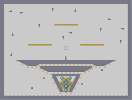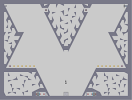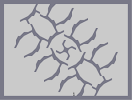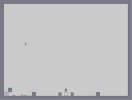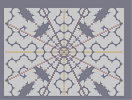A Very Small Race Super Smash Bros. Tribute 1 Climbing Mt. Everest Parasite Death DDA Supernova

Pages: (0)

### 4/5

Shadows of the letters 'S' are a bit failed, but the overall picture seems amazing.
Looks like WordArt, i liked it.

### no i cant..

ill go to edit, uncheck it and submit it and it will check itself again.. its probly pickin something up in the map data

4.5

4/5

4

5/5